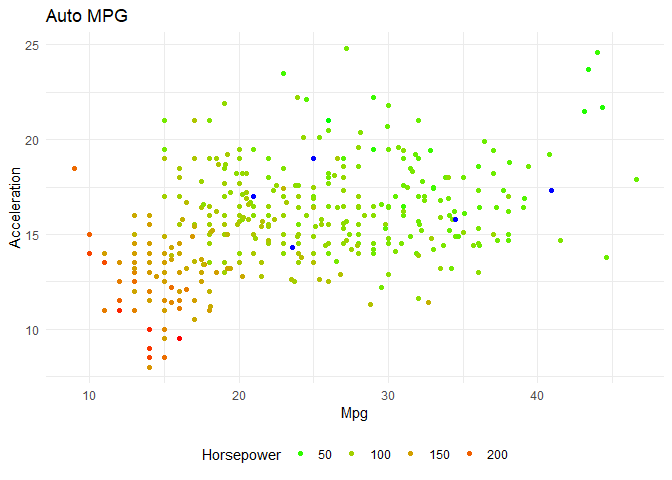# Null Value Imputation (R)

## Problem¶

Real world data is not always clean. Its often messy and contains unexpected/missing values. In this post I will use a non-parametric algorithm called k-nearest-neighbors (KNN) to replace missing values.

## Data¶

The data is technical spec of cars. I have taken this data set from UCI Machine learning repository which in turn took it from StatLib library which is maintained at Carnegie Mellon University. The data set was used in the 1983 American Statistical Association Exposition.

Sample Data
mpg cylinders displacement horsepower weight acceleration year origin name
20.0 6 156 122 2807 13.5 73 3 toyota mark ii
37.7 4 89 62 2050 17.3 81 3 toyota tercel
15.5 8 304 120 3962 13.9 76 1 amc matador
23.0 4 120 97 2506 14.5 72 3 toyouta corona mark ii (sw)
30.7 6 145 76 3160 19.6 81 2 volvo diesel

The data set contains the following columns:
1. mpg: continuous (miles per gallon)
2. cylinders: multivalued discrete 3. displacement: continuous (cu. inches)
4. horsepower: continuous
5. weight: continuous(lbs.)
6. acceleration: continuous (sec.)
7. model year: multivalued discrete (modulo 100)
8. origin: multivalued discrete (1. American, 2. European, 3. Japanese)
9. car name: string (unique for each instance)

Now I want to find if this data set contains any abnormal values.

``````summary(cars_info)
``````
``````##       mpg          cylinders      displacement     horsepower        weight
##  Min.   : 9.00   Min.   :3.000   Min.   : 68.0   Min.   : 46.0   Min.   :1613
##  1st Qu.:17.50   1st Qu.:4.000   1st Qu.:104.0   1st Qu.: 75.0   1st Qu.:2223
##  Median :23.00   Median :4.000   Median :146.0   Median : 93.5   Median :2800
##  Mean   :23.52   Mean   :5.458   Mean   :193.5   Mean   :104.5   Mean   :2970
##  3rd Qu.:29.00   3rd Qu.:8.000   3rd Qu.:262.0   3rd Qu.:126.0   3rd Qu.:3609
##  Max.   :46.60   Max.   :8.000   Max.   :455.0   Max.   :230.0   Max.   :5140
##                                                  NA's   :5
##   acceleration        year       origin      name
##  Min.   : 8.00   Min.   :70.00   1:248   Length:397
##  1st Qu.:13.80   1st Qu.:73.00   2: 70   Class :character
##  Median :15.50   Median :76.00   3: 79   Mode  :character
##  Mean   :15.56   Mean   :75.99
##  3rd Qu.:17.10   3rd Qu.:79.00
##  Max.   :24.80   Max.   :82.00
##
``````

## KNN¶

I find that horsepower contains 5 NA values. I can ignore the data points with horsepower NA, or I could impute the NA values using KNN or other methods. Before imputing, I want to make a strong case that my imputation would be right.

Cars with missing horsepower
mpg cylinders displacement weight acceleration year origin name
25.0 4 98 2046 19.0 71 1 ford pinto
21.0 6 200 2875 17.0 74 1 ford maverick
40.9 4 85 1835 17.3 80 2 renault lecar deluxe
23.6 4 140 2905 14.3 80 1 ford mustang cobra
34.5 4 100 2320 15.8 81 2 renault 18i

The assumption behind using KNN for missing values is that a point value can be approximated by the values of the points that are closest to it, based on other variables.
Let me take three variables from the above data set, mpg, acceleration and horsepower. Intuitively, these variables seem to be related.

``````ggplot(cars_info, aes(x = mpg, y = acceleration, color = horsepower)) +
geom_point(show.legend = TRUE) +
labs(x = 'Mpg', y='Acceleration',  title = "Auto MPG",
color = 'Horsepower') +
scale_color_gradient(low = "green", high = "red",
na.value = "blue", guide = "legend") +
theme_minimal()+theme(legend.position="bottom")
``````In the above plot, the blue colour points are null values. I can infer that cars of similar mpg and acceleration have similar horsepower. For a given missing value, I can look at the mpg of the car, its acceleration, look for its k nearest neighbours and get the car's horsepower.
I am using preprocess function in caret package for imputing NA's. The K value that I am taking is 20 (~ close to square root of number of variables)

## Imputation using caret¶

``````library(caret)
preProcValues <- preProcess(cars_info %>%
dplyr::select(mpg, cylinders, displacement, weight, acceleration, origin, horsepower),
method = c("knnImpute"),
k = 20,
knnSummary = mean)
impute_cars_info <- predict(preProcValues, cars_info,na.action = na.pass)
``````

The impute_cars_info data set will be normalized. To de-normalize and get the original data back:

``````procNames <- data.frame(col = names(preProcValues\$mean), mean = preProcValues\$mean, sd = preProcValues\$std)
for(i in procNames\$col){
impute_cars_info[i] <- impute_cars_info[i]*preProcValues\$std[i]+preProcValues\$mean[i]
}
``````

The imputed horsepower for the missing data points is:

Imputed data set
name year origin mpg cylinders displacement weight acceleration horsepower
ford maverick 74 1 21.0 6 200 2875 17.0 93.60
ford mustang cobra 80 1 23.6 4 140 2905 14.3 94.95
ford pinto 71 1 25.0 4 98 2046 19.0 72.45
renault 18i 81 2 34.5 4 100 2320 15.8 73.75
renault lecar deluxe 80 2 40.9 4 85 1835 17.3 65.10

The actual hp for the cars is as follows:

Comparison
name year horsepower actual_hp difference
ford maverick 74 93.60 84 9.60
ford mustang cobra 80 94.95 118 23.05
ford pinto 71 72.45 100 27.55
renault 18i 81 73.75 81 7.25
renault lecar deluxe 80 65.10 51 14.10

Out of the 5 cars, I was able to impute horsepower for 2 cars with less than 10hp difference, one car within 15hp and two cars within 30hp difference. To get better results, I should use other imputation techniques. Generally these 5 cars are removed while doing any analysis. In R, you could find the removed data set as mtcars.